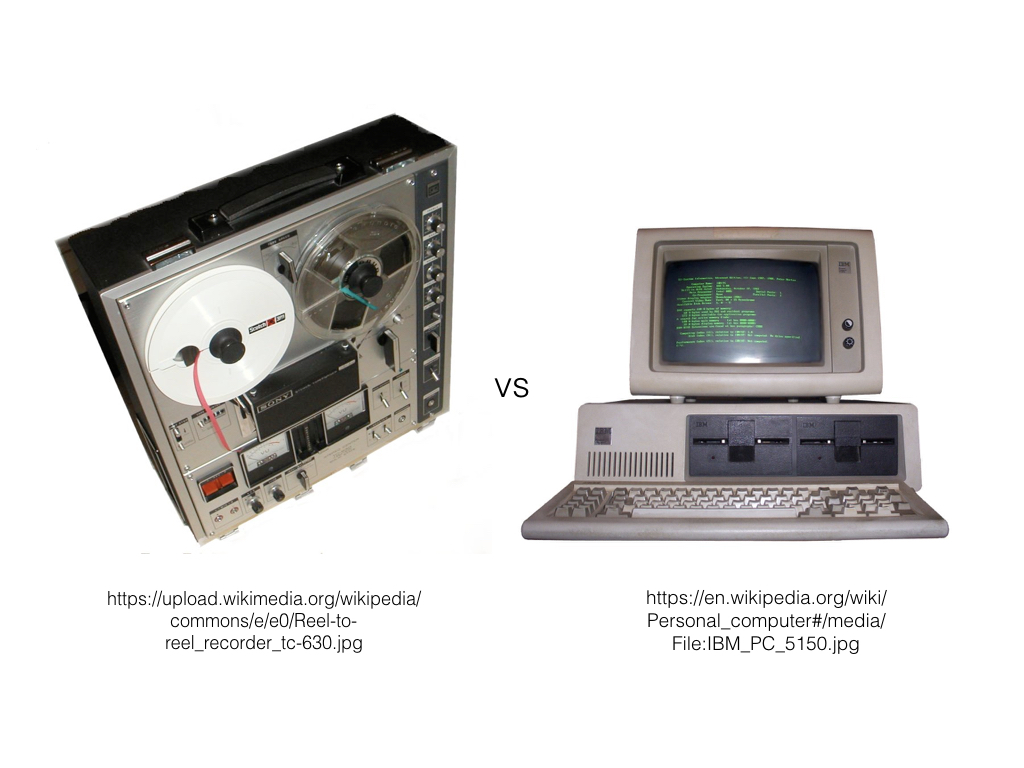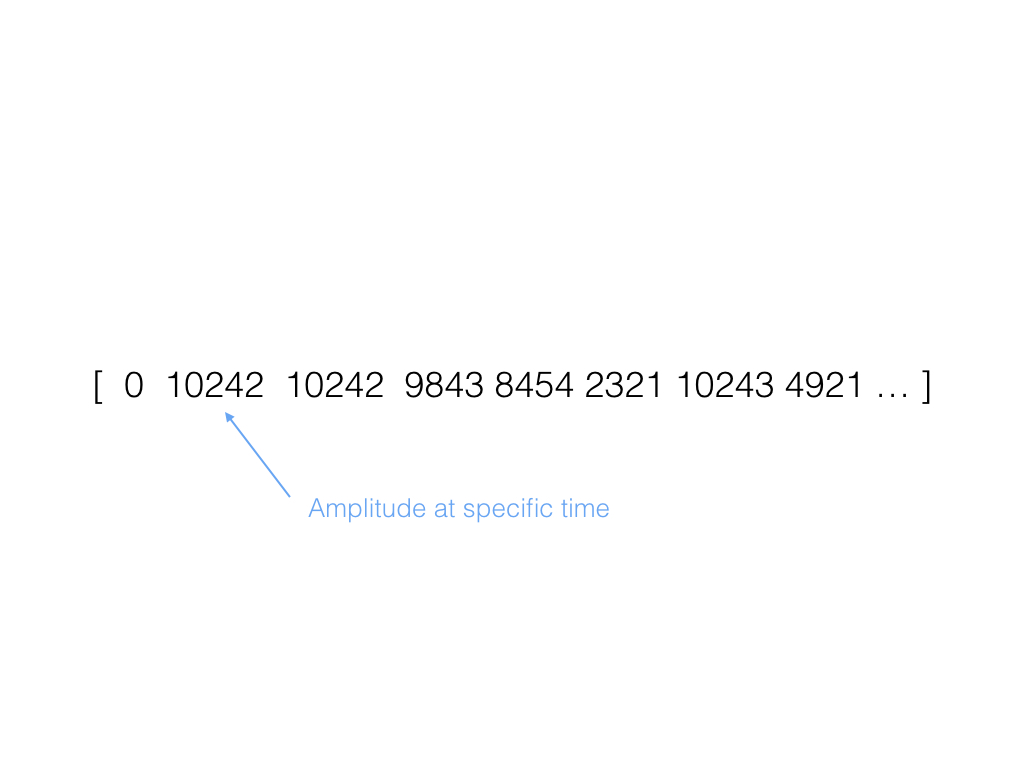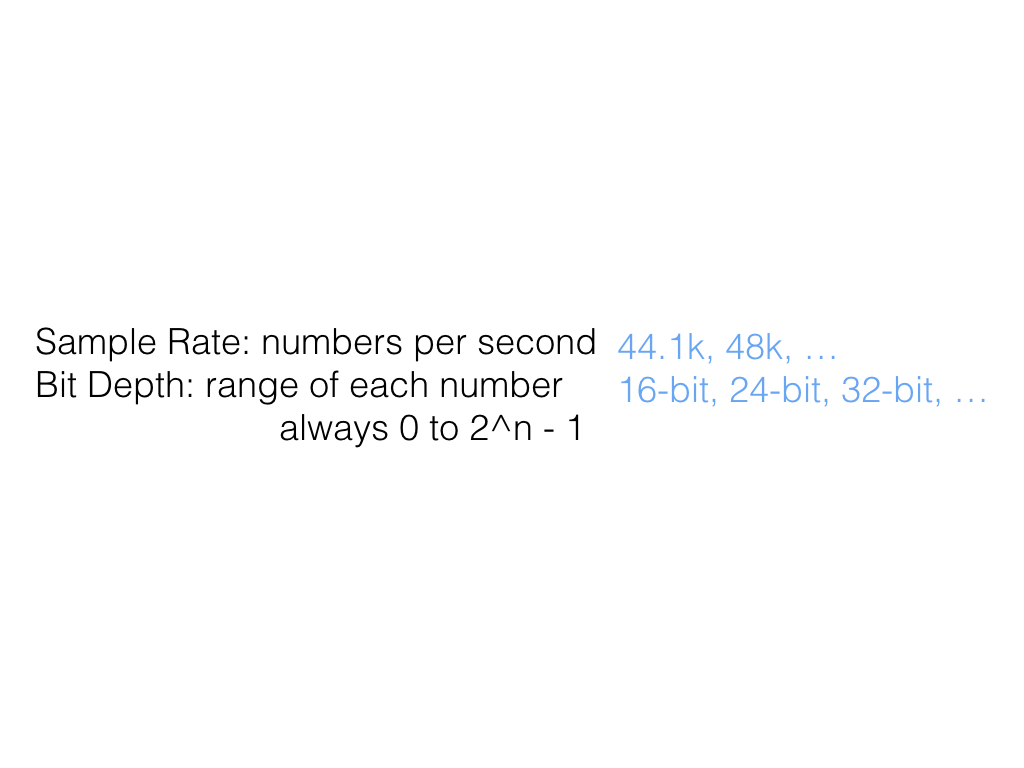Page Card

# Digital Representation

Belongs to subject Digital Representation

We represent digital sound quite differently than analog sound. While computers are impressive at doing arithmetic at lightning speeds, the computer must represent sound as numbers instead of physical signals.# Representation

The computer understands sound as a long series of large numbers. We often call this series an "array". Each number represents a specific amplitude for a very small amount of time.For album quality audio, each number represents 1 / 44,100th of a second! Each number can range from 0 to 65535. And in computer fashion, each number must be an exact integer.# Sample Rate

The amount of time each sample represents refers to the "sample rate". The most common sample rate is 44,100, also known as 44.1k. We also often use 48k and other rates. This rate is literally the amount of numbers per second.

# Bit DepthThe possible range of each number is the "bit depth". 16 bit numbers, or 0 to 65535, are the most common. 24 and 32 bit systems are becoming more regular. Computers often represent numbers in the range of 0 to 2 to the power of some value minus 1. 16 bits means 2 to the power of 16, and we subtract 1 at the top of the range to allow for 0.

44.1k sample rate and 16 bit depth is way more than sufficient for human listening capabilities. Sometimes we use faster sample rates and larger bit depths so when we modify sounds to the extreme, we don't drop below the needed amounts for human listening.